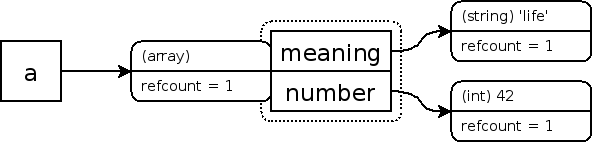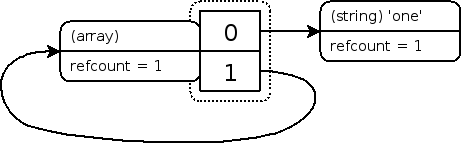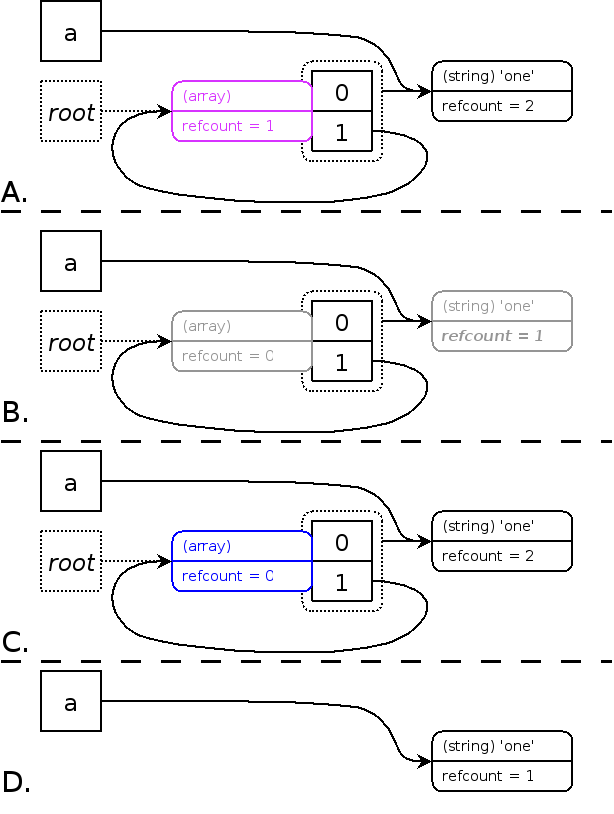# PHP 垃圾回收与内存管理指引

## 引用计数

### 引用计数基础知识

php 的变量存储在「zval」变量容器（数据结构）中，「zval」属性包含如下信息：

• 当前变量的数据类型；
• 当前变量的值；
• 用于标识变量是否为引用传递的 is_ref 布尔类型标识；
• 指向该「zval」变量容器的变量个数的 refcount 标识符（即这个 zval 被引用的次数，注意这里的引用不是指引用传值，注意区分）。

### 查看变量 zval 容器信息

• 查看普通变量的 zval 信息

``````<?php
// 变量赋值时，refcount 值等于 1
\$name = 'liugongzi';
xdebug_debug_zval('name'); // (refcount=1, is_ref=0)string 'liugongzi' (length=9)

// \$name 作为值赋值给另一个变量， refcount 值增加 1
\$copy = \$name;
xdebug_debug_zval('name'); // (refcount=2, is_ref=0)string 'liugongzi' (length=9)

// 销毁变量，refcount 值减掉 1
unset(\$copy);
xdebug_debug_zval('name'); // (refcount=1, is_ref=0)string 'liugongzi' (length=9)``````
• 写时复制

``````<?php
\$name = 'liugongzi';
xdebug_debug_zval('name'); // name: (refcount=1, is_ref=0)string 'liugongzi' (length=9)

\$copy = \$name;
xdebug_debug_zval('name'); // name: (refcount=2, is_ref=0)string 'liugongzi' (length=9)

// 将新的值赋值给变量 \$copy
\$copy = 'liugongzi handsome';
xdebug_debug_zval('name'); // name: (refcount=1, is_ref=0)string 'liugongzi' (length=9)
xdebug_debug_zval('copy'); // copy: (refcount=1, is_ref=0)='liugongzi handsome'``````

• 将 \\$copy 从 \\$name 的 zval（内从）中分离出来（即复制）；
• 将 \\$name 的 refcount 减去 1；
• 对 \\$copy 的 zval 进行修改（重新赋值和修改 refcount）；

• 查看引用传递变量的 zval 信息

``````<?php
\$age = 'liugongzi';
xdebug_debug_zval('age'); // (refcount=1, is_ref=0)string 'liugongzi' (length=9)

\$copy = &\$age;
xdebug_debug_zval('age'); // (refcount=2, is_ref=1)string 'liugongzi' (length=9)

unset(\$copy);
xdebug_debug_zval('age'); // (refcount=1, is_ref=1)string 'liugongzi' (length=9)``````
• 复合类型的引用计数

``````\$a = array( 'meaning' => 'life', 'number' => 42 );
xdebug_debug_zval( 'a' );

// a:
// (refcount=1, is_ref=0)
// array (size=2)
//  'meaning' => (refcount=1, is_ref=0)string 'life' (length=4)
//  'number' => (refcount=1, is_ref=0)int 42````````````\$a = array( 'meaning' => 'life', 'number' => 42 );
xdebug_debug_zval( 'a' );
\$a['life'] = \$a['meaning'];
xdebug_debug_zval( 'a' );

// a:
// (refcount=1, is_ref=0)
// array (size=3)
//  'meaning' => (refcount=2, is_ref=0)string 'life' (length=4)
//  'number' => (refcount=0, is_ref=0)int 42
//  'life' => (refcount=2, is_ref=0)string 'life' (length=4)``````• 内存泄露

``````<?php
function convert(\$size)
{
\$unit=array('b','kb','mb','gb','tb','pb');
return @round(\$size/pow(1024,(\$i=floor(log(\$size,1024)))),2).' '.\$unit[\$i];
}

// 注意：有用的地方从这里开始
\$memory = memory_get_usage();

\$a = array( 'one' );

// 引用自身（循环引用）
\$a[] =&\$a;

xdebug_debug_zval( 'a' );

var_dump(convert(memory_get_usage() - \$memory)); // 296 b

unset(\$a); // 删除变量 \$a，由于 \$a 中的元素引用了自身（循环引用）最终导致 \$a 所使用的内存无法被回收

var_dump(convert(memory_get_usage() - \$memory)); // 568 b``````## 引用计数系统的同步周期回收

• 引入了可能根（possible root）的概念：通过引用计数相关学习，我们知道如果一个变量（zval）被引用，要么是被全局符号表中的符号引用（即变量），要么被复杂类型（如数组）的 zval 中的符号（数组的元素）引用，那么这个 zval 变量容器就是「可能根」。
• 引入根缓冲区（root buffer）的概念：根缓冲区用于存放所有「可能根」，它是固定大小的，默认可存 10000 个可能根，如需修改可以通过修改 PHP 源码文件 Zend/zend_gc.c 中的常量 GC_ROOT_BUFFER_MAX_ENTRIES，再重新编译。
• 回收周期：当缓冲区满时，对缓冲区中的所有可能根进行垃圾回收处理。### 引用计数系统的同步周期回收过程

1. 缓冲区（紫色框部分，称为疑似垃圾），存储所有可能根（步骤 A）；
2. 采用深度优先算法遍历「根缓冲区」中所有的「可能根（即 zval 遍历容器）」，并对每个 zval 的 refcount 减 1，为了避免遍历时对同一个 zval 多次减 1（因为不同的根可能遍历到同一个 zval）将这个 zvel 标记为「已减」（步骤 B）；
3. 再次采用深度优先遍历算法遍历「可能根 zval」。当 zval 的 refcount 值不为 0 时，对其加 1,否则保持为 0。并请已遍历的 zval 变量容器标记为「已恢复」（即步骤 B 的逆运算）。那些 zval 的 refcount 值为 0 （蓝色框标记）的就是应该被回收的变量（步骤 C）；
4. 删除所有 refcount 为 0 的可能根（步骤 D）。

### 优化后的引用计数算法优势

• 将内存泄露控制在阀值内，这个由缓存区实现，达到缓冲区大小执行新一轮垃圾回收；
• 提升了垃圾回收性能，不是每次 refcount 减 1 都执行回收处理，而是等到根缓冲区满时才开始执行垃圾回收。

## PHP 7 的内存管理

PHP 5 中 zval 实现上的主要问题：

• zval 总是单独 从堆中分配内存；
• zval 总是存储引用计数和循环回收 的信息，即使是整型（bool / null）这种可能并不需要此类信息的数据；
• 在使用对象或者资源时，直接引用会导致两次计数；
• 某些间接访问需要一个更好的处理方式。比如现在访问存储在变量中的对象间接使用了四个指针（指针链的长度为四）；
• 直接计数也就意味着数值只能在 zval 之间共享。如果想在 zval 和 hashtable key 之间共享一个字符串就不行（除非 hashtable key 也是 zval）。

PHP 7 中的 zval 数据结构实现的调整：

• 简单数据类型不需要单独分配内存，也不需要计数；
• 不会再有两次计数的情况。在对象中，只有对象自身存储的计数是有效的；
• 由于现在计数由数值自身存储（PHP 有 zval 变量容器存储），所以也就可以和非 zval 结构的数据共享，比如 zval 和 hashtable key 之间；
• 间接访问需要的指针数减少了。

## 参考资料

TPIP：第六节 写时复制（Copy On Write）

TPIP：内存管理

PHP7 内核之 zval

Confusion about PHP 7 refcount

PHP7 革新与性能优化